Latest Banking jobs   »

# Reasoning Ability Quiz For LIC ADO Mains 2023-20th March

Directions (1-5): The questions below consist of two statements numbered I and II given below it. You have to decide whether the data provided in the statements are sufficient to answer the question. Read all the statements and answer the question.

Q1. Six persons I, J, K, L, M, and N have different heights. Who is the tallest among them?
Statements:
I: Two persons are shorter than J. I is taller than K but neither of them is shorter than J. L is taller than M who is not the shortest. Neither K nor L is 2nd tallest person.
II: I is taller than J and M. Only N is shorter than M. L is taller than K who is the 3rd tallest person.
(a) Data given in both statements I and II together are sufficient to answer.
(b) Data given in statement I alone is sufficient to answer.
(c) Data given in statement II alone is sufficient to answer.
(d) Data given in both statements I and II together are not sufficient to answer.
(e) Data given in either statement I or statement II alone is sufficient to answer the question.

Q2. Seven persons P, Q, R, S, T, U and V have an exam on seven different days starting from Monday to Sunday in the same week. No two persons have exam on same day. Who among the given person has exam on Friday?
Statements:
I: Only two persons have exam between the exams of P and Q whose exam is not on Monday. Only one person has the exam between P and R.
II: U’s exam is neither on Thursday nor on Saturday but U has exam on two days before the exam of V.
(a) Data given in both statements I and II together are sufficient to answer.
(b) Data given in statement I alone is sufficient to answer.
(c) Data given in statement II alone is sufficient to answer.
(d) Data given in both statements I and II together are not sufficient to answer.
(e) Data given in either statement I or statement II alone is sufficient to answer the question.

Q3. Eight boxes P, Q, R, S, T, U, V and W are kept one above the other like a stack. The bottommost box is numbered 1 and the topmost box is numbered 8. How many boxes are between U and W?
Statements:
I: Q is three boxes above R. V is immediately above Q but not the 8th box. Four boxes are below P. U is three boxes above T.
II: W is three boxes above P which is above U. V is immediately above Q which is not 5th box. U is two boxes above S.
(a) Data given in both statements I and II together are sufficient to answer.
(b) Data given in statement I alone is sufficient to answer.
(c) Data given in statement II alone is sufficient to answer.
(d) Data given in both statements I and II together are not sufficient to answer.
(e) Data given in either statement I or statement II alone is sufficient to answer the question.

Q4. In the given coded language, how is the word “Acquire” coded?
Statements:
I: ‘going to acquire medal’ is coded as cp, pc, mr, cd’ and ‘medal for our india’ is coded as pc, km, dc, rm’.
II: ‘he acquire many medal’ is coded as ‘pc, mk, cp, rg’ and ‘going and winning medal’ is coded as ‘rd, pc, ug, cd’.
(a) Data given in both statements I and II together are sufficient to answer.
(b) Data given in statement I alone is sufficient to answer.
(c) Data given in statement II alone is sufficient to answer.
(d) Data given in both statements I and II together are not sufficient to answer.
(e) Data given in either statement I or statement II alone is sufficient to answer the question.

Q5. Six persons A, B, C, D, E and F sit in a row facing north direction. Who among the following sit immediate left of F?
Statements:
I. E sits second to the left of A. C sits at one of the extreme ends. B is not adjacent to F.
II. B sits third to the left of A. Neither B nor A sit at extreme end. E sits immediate left of D. C sits to the left of F.
(a) Data given in both statements I and II together are sufficient to answer.
(b) Data given in statement I alone is sufficient to answer.
(c) Data given in statement II alone is sufficient to answer.
(d) Data given in both statements I and II together are not sufficient to answer.
(e) Data given in either statement I or statement II alone is sufficient to answer the question.

Directions (6-9): The questions below consist of two statements numbered I and II given below it. You have to decide whether the data provided in the statements are sufficient to answer the question. Read all the statements and answer the question.

Q6. Seven persons M, N, O, P, Q, R and S live on different floors of a building. The bottommost floor is numbered as 1, floor above it as 2 and so on. Who lives on 4th floor?
Statements:
I. R lives three floors above S who lives on prime numbered floor. P lives on 2nd floor. N lives just above M.
II. Two persons live above N’s floor. S lives just above P who lives just above O. M lives below N but not on bottommost floor.
(a) Data given in both statements I and II together are sufficient to answer.
(b) Data given in statement I alone is sufficient to answer.
(c) Data given in statement II alone is sufficient to answer.
(d) Data given in both statements I and II together are not sufficient to answer.
(e) Data given in either statement I or statement II alone is sufficient to answer the question.

Q7. Six persons, M, N, O, P, Q and R are sitting around a circular table and all of them are facing the centre. Who is sitting 2nd to the right of O?
Statements:
I: P is sitting 2nd to the left of N. R is sitting adjacent to P. Q is sitting 2nd to the left of R.
II: O is not sitting adjacent to N. M is not sitting opposite to P.
(a) Data given in both statements I and II together are sufficient to answer.
(b) Data given in statement I alone is sufficient to answer.
(c) Data given in statement II alone is sufficient to answer.
(d) Data given in both statements I and II together are not sufficient to answer.
(e) Data given in either statement I or statement II alone is sufficient to answer the question.

Q8. What is the code for ‘switch’ in a certain code language?
Statements:
I: In a certain code of language, ‘switch the ways technique now’ is coded as ‘bp mp kp ip op’ and ‘ways are easy than’ is coded as ‘kp op hp qp
II: In a certain code of language, ‘switch the places than’ is coded as ‘mp ap ip qp’ and ‘technique products quality’ is coded as ‘bp up yp’
(a) Data given in both statements I and II together are sufficient to answer.
(b) Data given in statement I alone is sufficient to answer.
(c) Data given in statement II alone is sufficient to answer.
(d) Data given in both statements I and II together are not sufficient to answer.
(e) Data given in either statement I or statement II alone is sufficient to answer the question.

Q9. Five persons, O, P, Q, M and N are ranked on the basis of the number of cards they bought. Who among the given person bought the least number of cards?
Statements:
I: O bought more number of cards than M and less number of cards than P. Neither Q nor N bought the highest number of cards.
II: Q bought less number of cards than only two persons. O bought less number of cards than N and P but not the least number of cards.
(a) Data given in both statements I and II together are sufficient to answer.
(b) Data given in statement I alone is sufficient to answer.
(c) Data given in statement II alone is sufficient to answer.
(d) Data given in both statements I and II together are not sufficient to answer.
(e) Data given in either statement I or statement II alone is sufficient to answer the question.

Directions (10-12): The question below consists of a question and three statements numbered I, II and III given below it. You have to decide whether the data provided in the statements are sufficient to answer the question. Read all the statements and give answer.

Q10. What is the code for ‘remote action’ in the given code language?
Statements:
I. If “confidence speed and remote’ is coded as, ‘nr, da, ar, li’
II. If ‘remote action are useful’ is coded as, ‘nr, mo, pr, gd’
III. If ‘action solutions and speed’ is coded as, ‘da, mo, ar, pd’
(a) Data given in only statements I and II are together sufficient to answer.
(b) Data given in only statements II and III are together sufficient to answer.
(c) Data given in only statements I and III are together sufficient to answer.
(d) Data given in all three statements I, II and III are together sufficient to answer.
(e) Data given in all three statements I, II and III together are not sufficient to answer.

Q11. Eight boxes M, N, O, P, Q, R, S and T are kept one above another but not necessarily in the same order. The bottommost box is numbered as 1 and the topmost box is numbered as 8. How many boxes are kept between Box M and Box R?
Statements:
I. Only three boxes are kept below Box N. Three boxes are kept between Box N and Box T. Box R is kept at one of the places above Box N.
II. Only three boxes are kept between Box Q and Box R. Only one box is kept between Box R and Box P.
III. Box M is not kept above Box P. Three boxes are kept between Box O and Box P.
(a) Data given in only statements I and II are together sufficient to answer.
(b) Data given in only statements II and III are together sufficient to answer.
(c) Data given in only statements I and III are together sufficient to answer.
(d) Data given in all three statements I, II and III are together sufficient to answer.
(e) Data given in all three statements I, II and III together are not sufficient to answer.

Q12. Eight persons A, B, C, D, T, U, V and W are born on 2nd in the different months from January to August in the same year. Who is the youngest among them?
Statements:
I. Two persons were born between T and U, who was born immediately after W.
II. Three persons were born between W and A, who was born after W.
III. D was born before W but after V.
(a) Data given in only statements I and II are together sufficient to answer.
(b) Data given in only statements II and III are together sufficient to answer.
(c) Data given in only statements I and III are together sufficient to answer.
(d) Data given in all three statements I, II and III are together sufficient to answer.
(e) Data given in all three statements I, II and III together are not sufficient to answer.

Directions (13-15): The questions below consist of two statements numbered I and II given below it. You have to decide whether the data provided in the statements are sufficient to answer the question. Read all the statements and answer the question.

Q13. Nine persons B, C, D, E, F, G, H, I and J sit in a straight row facing north. No two persons with names starting with consecutive alphabets as per English Alphabetical order sit adjacent to each other. How many persons sit H and 1?
Statements:
I: G sits 3rd to the left of C. H sits to the left of G. Only E and I sit to the right of C
II: H sits to the left of F. C sits to the right of B. More than two persons sit to the left of I.
(a) Data given in both statements I and II together are sufficient to answer.
(b) Data given in statement I alone is sufficient to answer.
(c) Data given in statement II alone is sufficient to answer.
(d) Data given in both statements I and II together are not sufficient to answer.
(e) Data given in either statement I or statement II alone is sufficient to answer the question.

Q14. Eight persons A, B, C, D, E, F, G, and H are sitting around a circular table facing the centre. Who sits immediate left of F?
Statements:
I. G does not sit adjacent to F. A sits third to the left of F who sits 3rd to the left of C.
II. Three persons sit between D and C who sits immediate right of E.
(a) Data given in both statements I and II together are sufficient to answer.
(b) Data given in statement I alone is sufficient to answer.
(c) Data given in statement II alone is sufficient to answer.
(d) Data given in both statements I and II together are not sufficient to answer.
(e) Data given in either statement I or statement II alone is sufficient to answer the question.

Q15. Six persons M, N, O, P, Q and R live on different floors of a 8-storay building. Two of them like different colors (Green and Blue). The bottommost floor is numbered 1 and the topmost floor as numbered 5. How many persons live between M and O?
Statements:
I. Persons who likes colors, lives on prime numbered floor. M lives at least two floors above O. P likes blue color and lives above O.
II. Q lives three floors above the one who likes Blue. One person lives between Q and N. Person who likes green, lives two floors above P who lives above 2nd floor.
(a) Data given in both statements I and II together are sufficient to answer.
(b) Data given in statement I alone is sufficient to answer.
(c) Data given in statement II alone is sufficient to answer.
(d) Data given in both statements I and II together are not sufficient to answer.
(e) Data given in either statement I or statement II alone is sufficient to answer the question.

Solutions

Solution (1-5):
S1. Ans. (b)
Sol. From statement I alone:
L > I > K > J > M > N
Hence, data given in statement I alone is sufficient to answer.

S2. Ans. (d)
Sol. From both statements, we can’t get the answer. So, data given in both statements I and II together are not sufficient to answer.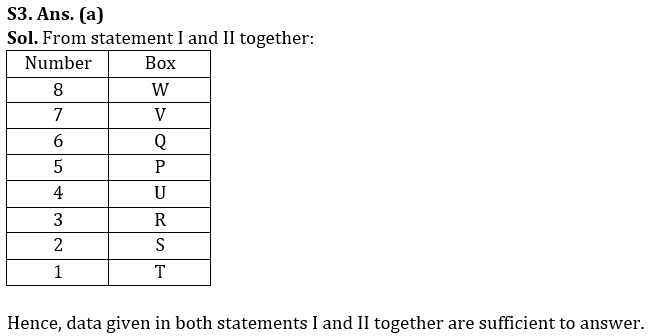S4. Ans. (a)
Sol. From statement I and II together, we find that “acquire” is coded as “cp”. So, data given in both statements I and II together are sufficient to answer.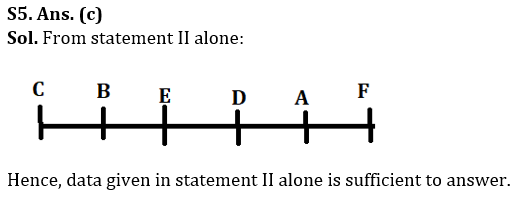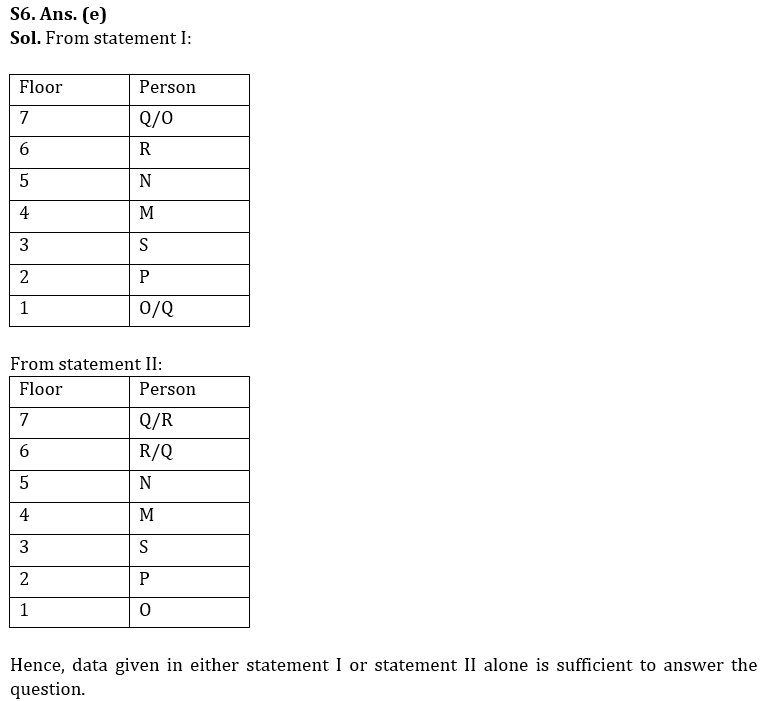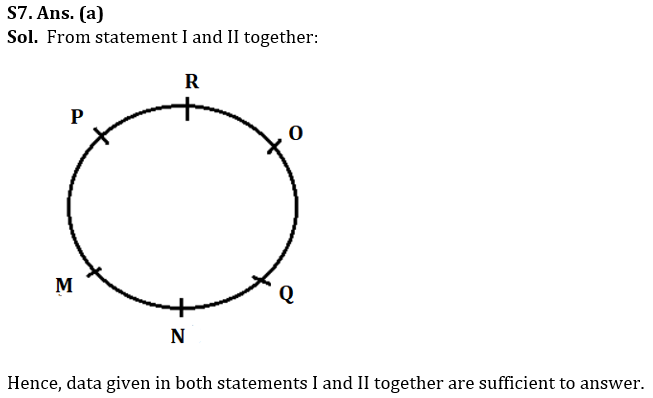S8. Ans. (d)
Sol. From both statements, we can’t get the answer. So, data given in both statements I and II together are not sufficient to answer.

S9. Ans. (c)
Sol. From statement II alone:
P/N > N/P > Q > O > M
Hence, data given in statement II alone is sufficient to answer.

Solution (10-12):
S10. Ans. (d)
Sol. From statement I, II, and III we get the code for ‘remote action’ as ‘nr mo’. Hence, Data given in all three statements I, II and III are together sufficient to answer.

S11. Ans. (e)
Sol. From all statements together, we can’t get the answer. So, data given in both statements I, II and III together are not sufficient to answer.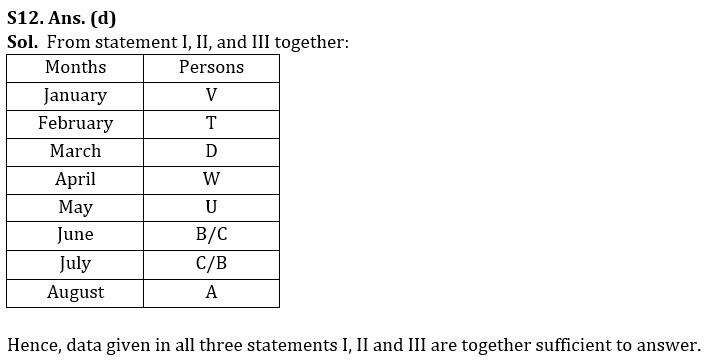Solution (13-15):
S13. Ans. (d)
Sol. From both statements, we can’t get the answer. So, data given in both statements I and II together are not sufficient to answer.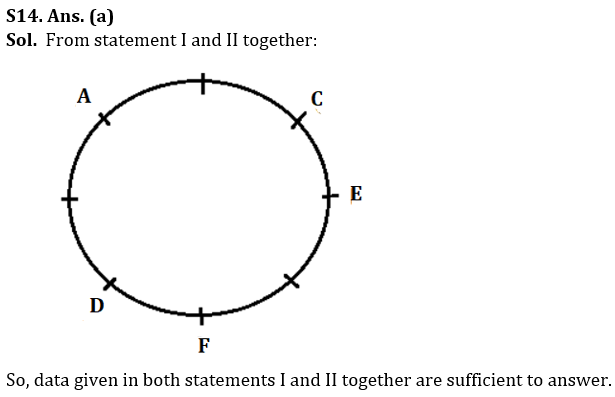S15. Ans. (d)
Sol. From both statements, we can’t get the answer. So, data given in both statements I and II together are not sufficient to answer.## FAQs

### When is the LIC ADO Mains Exam scheduled?

The LIC ADO Mains exam is scheduled to be held on 23 April 2023

#### Congratulations!Union Budget 2023-24: Free PDF